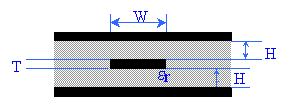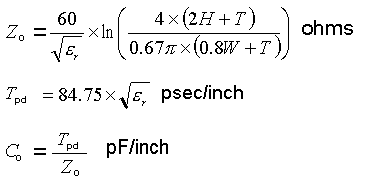### Centered Stripline Impedance CalculatorProvide values for three of the four parameters H, T, W, Zo and the relative permittivity of the dielectric. Click the button corresponding to the unknown parameter to calculate its value. ( W/(H-T) < 0.35; T/H <0.25; 1 < εr < 15 )

Select the units for all dimensions: mm mils
 (Thickness):mm mils (Trace thickness):mm mils (Trace width):mm mils εr (Relative permittivity):(Characteristic impedance):ohms C0   (Capacitance):pF/cm pF/inch Tpd  (Propagation delay time):psec/cm psec/inch

#### Notes

• The formulas used here are taken from the Design Guide for Electronic Packaging Utilizing High-Speed Techniques (4th Working Draft, IPC-2251, February 2001).• Range of valid parameters specified in the Design Guide: W/(H-T) < 0.35; T/H <0.25; 1 < εr < 15
• Accuracy: For typical PCB parameters (εr = 4, H = 15 mil and T = 1.37 mil), the deviation of the calculated results from results obtained using a 2D numerical field solver, is listed below:  Z0: W/(H-T) < 0.05 more than 30% 0.05 < W/(H-T) < 0.08 within 2% 0.08 < W/H < 0.32 within 1% 0.32 < W/(H-T) < 0.35 within 2% C0: W/(H-T) < 0.05 more than 100% 0.05 < W/(H-T) < 0.08 within 2% 0.08 < W/H < 0.32 within 1% 0.32 < W/(H-T) < 0.35 within 2%